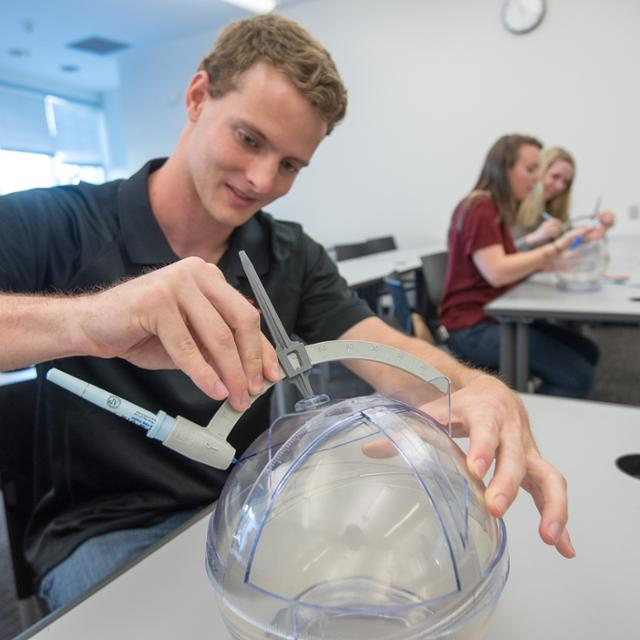# BS in Actuarial ScienceThis is the more demanding of the two undergraduate majors offered in the Department of Mathematics. This degree is appropriate for students planning to go on to graduate school in mathematics and related fields, and for anyone who wants a more challenging mathematical experience at TCU. The actuarial concentration is specifically designed to prepare students for careers in insurance, risk analysis, pension management, financial planning and other related areas.

This degree requires 46 semester hours of courses in mathematics.

Students must take the following 31 hours of mathematics courses:

• MATH 10524 Calculus I
• MATH 20123 Discrete Mathematics I
• MATH 20524 Calculus II
• MATH 30053 Intro to Mathematical Proof
• MATH 30224 Linear Algebra
• MATH 30524 Calculus III
• MATH 30603 Interest Theory
• MATH 30623 Interest Theory II
• MATH 30803 Probability
• MATH 30853 Statistics
• MATH 40603 Actuarial Mathematics

Students must also take one of the following programming courses:

• COSC 10403 Introduction to Programming
• COSC 10503 Introduction to Programming for Engineering and Science
• COSC 10603 Introduction to Python for Data Analytics
• ENGR 10573 Applied Programming Matlab

In addition, you must take two of the following courses:

• PHYS 20474 Physics I
• PHYS 20484 Physics II
• COSC 20203 Techniques in Programming
• ECON 31223 Intermediate Microeconomics: A Mathematical Approach
• ECON 40313 Econometrics

• ECON 10223 Introductory Microeconomics
• ECON 10233 Introductory Macroeconomics
• ECON 30223 Intermediate Microeconomics or ECON 31223 Intermediate Microeconomics: A Mathematical Approach
• ECON 30233 Intermediate Macroeconomics
• ACCT 20353 Fundamentals of Accounting
• ACCT 40163 Accounting for Decision Making and Control
• FINA 30153 Financial Management

Note that ECON 31223 can be applied to satisfy associated requirements from two of the above lists.

Students pursuing a BS degree in mathematics with an actuarial concentration have a choice of two tracks:

## Track 1

Students in this track must take both of the following courses:

• MATH 50253 Abstract Algebra I
• MATH 50503 Real Analysis I

The student also must take an additional three hours of mathematics courses at or above the 30000 level.

## Track 2

Students in this track must take one of the following courses:

• MATH 40223 Applied Linear Algebra
• MATH 40663 Numerical Analysis
• MATH 40853 Regression and Time Series
• MATH 40883 Predictive Modeling

The student also must take an additional six hours of mathematics course at or above the 30000 level; these six hours may include taking extra courses from the list of four options above.

All actuarial students need to work closely with an adviser to plan course schedules.

Credit is not allowed for both MATH 10283 and MATH 10524.

Students must earn a grade of C- or better in each mathematics course for that course to count toward a mathematics degree. Students must also have a 2.0 average or better in their mathematics courses in order to graduate with a degree in mathematics.

Students pursuing a program leading to a Bachelor of Science degree must complete a minimum of 124 semester hours, 42 of which must be advanced (30000 level or above) from TCU. In addition, students must complete the TCU Core Curriculum.